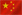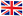中文|EN

# 5阶灰度测试卡

• 品牌：ESSER爱莎/Sine Image
型号：TE109
尺寸：可定制
材料：菲林/高清相纸
比例：4:3
类型：反射式/透射式可选
• Two 5-graduated counter current gray scales are arranged on a gray background (D=0.83), the gray scales being graduated logarithmically. Related to the densities of the gray scales: gamma= 0.45. Related to the remission values (brightness): gamma = 2.2, that being exactly the reciprocal value of gamma = 0.45.

The signal of the background is in the middle of the 3 rd and the 4th step. The output of an optimally gamma-corrected camera yields two 5-graduated counter current linear step signals. The contrast range of the gray scales is 40: 1.

The values of the 5-graduated gray scale are as follows:

 Step Density Reflectance in % Output voltage in % 1 0.15 71 100 2 0.37 43 77.5 3 0.65 22 55 4 1.05 9 32.5 5 1.75 2 10

The density values are based on a density of BaO4S=0. A field of black velvet is located between the gray scales. The density of this field is D > 2.4 (reflectance < 0.5 %).

RECOMMENDED PRODUCT